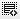## How to calculate avarage in Excel0
how to calculate avarage of a set of number in a colomn in one step0

Average is calculated by adding a group of numbers and then dividing by the count of those numbers. For example, the average of 2, 3, 3, 5, 7, and 10 is 30 divided by 6, which is 5.

To calculate the average, we use the AVERAGE function.
=AVERAGE(A1:A5)

This will calculate the average of the numbers in cells A1 to A5.

Click on thisicon to add code snippet.